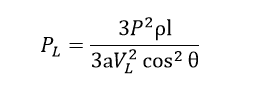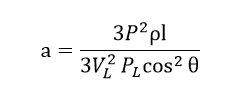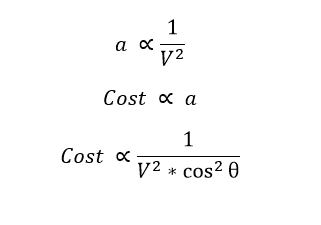# Why High Voltage is Preferred to Transmit the Power

### Why High Voltage is Preferred to Transmit the Power:

You can see there will be big size transmission tower will be installed nearer to the home or outside city with big size insulator, conductor, substation etc. all the transmission line is rated in kV because where high voltage is transmitted. Let see why high voltage transmission is preferred.

Reducing the size of the conductor and saves conductor cost:

Three phase power P,

VL= Line voltage

IL=Line current

Cosθ = Power factor

PL = Power losses

R= resistance.

ρ= resistivity of the conductor.

l= length of the conductor

a= Area of cross section

Three phase power P=√3 VL IL cosθ

From that current IL = P/√3 VL cosθ

Power losses in the conductor PL = 3 IL2 R

Here the resistance R=ρl/A

Hence the total power losses in the transmission lineHence cross section a is equal tohere you can see that, the cross section is indirectly proportional to the Voltage as the voltage increase the cross section also decrease.As we can conclude that, by increasing the voltage in the conductor significantly decrease the size of the conductor and results we get the same in reduction cost.

Reducing the Power loss:

IL = P/√3 VL cosθ, here the Line current is inversely proportional to the voltage, Also the line current proportional to the power losses, therefore increasing the voltage we can decreasing the current flow through the conductor. The current flow reduces the I^2 *R loss.

Reduction in percentage voltage Drop:

Line drop = IR= I * ρl/A = J * ρl

%line drop = J ρl/VL *100 here J and ρ are constant

As we increase the voltage, the % line drop decrease as well.

Improvement of Voltage regulation: When the voltage drops decreases, the voltage regulation also increases.

Increases the transmission efficiency

Bulk Power Transmission is possible

### Disadvantage of High voltage transmission line:

• Increases corona effect and radio interference. In high voltage line the potential gradient is high, therefore the high voltage transmission line creates high electromagnetic inference in communication line
• Line erection and commission cost is high
• Unsafe for living things
• Insulation and maintenance cost is very high.
• Fault in the single line causes tripping of all neighboring lines because in long transmission line the fault current in high.
• Needs high voltage special designed equipments for handing these high voltage lines.